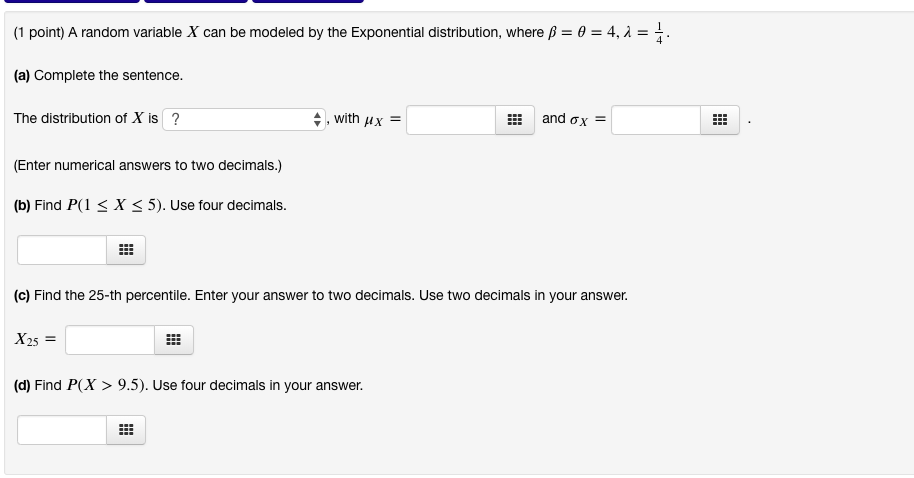KBS71G The Asker · Probability and StatisticsTranscribed Image Text: -4,2- (1 point) A random variable X can be modeled by the Exponential distribution, where ß- (a) Complete the sentence. The distribution of X is ? , with Hx and σχ- (Enter numerical answers to two decimals.) (b) Find P(1 < X < 5). Use four decimals. (c) Find the 25-th percentile. Enter your answer to two decimals. Use two decimals in your answer. X25 (d) Find P(X > 9.5). Use four decimals in your answer
More
Transcribed Image Text: -4,2- (1 point) A random variable X can be modeled by the Exponential distribution, where ß- (a) Complete the sentence. The distribution of X is ? , with Hx and σχ- (Enter numerical answers to two decimals.) (b) Find P(1 < X < 5). Use four decimals. (c) Find the 25-th percentile. Enter your answer to two decimals. Use two decimals in your answer. X25 (d) Find P(X > 9.5). Use four decimals in your answer